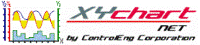XY Chart NET 3 Control Reference
NULL Datapoints

NULL Datapoints feature allows you to omit certain data points from being plotted on the chart by setting its value to NULL (1.79E+308).  All data points with a value of 1.79E+308 will not be displayed on the chart.

To Enable Feature
You can use the built in SetNULLData method, along with Row and Column properties, to set a datapoint to NULL (see example 1).  You can also manually set a datapoint to NULL by setting Row, Column, and Data properties (see example 2).  Examples 1 and 2 are functionally identical.
Example 1
```' XYChartNETCtl1 is the name of the XY Chart NET control instance placed on the form.

With XYChartNETCtl1
.NumProfiles = 3             'Set the number of profiles to plot to 3
.Profile(0).NumSamples = 10  'Set the number of samples to 10
.Profile(1).NumSamples = 10  'Set the number of samples to 10
.Profile(2).NumSamples = 10  'Set the number of samples to 10

.Column = 5                  'Set the column to 5, i.e. Y-data for Profile 2
.Row = 2                     'Set row to 2, i.e. 3rd sample Y-data for Profile 2
.SetNULLData()               'Set the data at (Row, Column) cell location to NULL

.Refresh                     'Refresh required to update chart with changes made
End With
```
```// XYChartNETCtl1 is the name of the XY Chart NET control instance placed on the form.

XYChartNETCtl1.NumProfiles = 3;           //Set the number of profiles to plot to 3
XYChartNETCtl1.get_Profile(0).NumSamples = 10;  //Set the number of samples to 10
XYChartNETCtl1.get_Profile(1).NumSamples = 10;  //Set the number of samples to 10
XYChartNETCtl1.get_Profile(2).NumSamples = 10;  //Set the number of samples to 10

XYChartNETCtl1.Column = 5;                //Set the column to 5, i.e. Y-data for Profile 2
XYChartNETCtl1.Row = 2;                   //Set row to 2, i.e. 3rd sample Y-data for Profile 2
XYChartNETCtl1.SetNULLData();             //Set the data at (Row, Column) cell location to NULL
XYChartNETCtl1.Refresh();                 //Refresh required to update chart with changes made
```
```// XYChartNETCtl1 is the name of the XY Chart NET control instance placed on the form.

XYChartNETCtl1->NumProfiles = 3;              //Set the number of profiles to plot to 3
XYChartNETCtl1->get_Profile(0)->NumSamples = 10;  //Set the number of samples to 10
XYChartNETCtl1->get_Profile(1)->NumSamples = 10;  //Set the number of samples to 10
XYChartNETCtl1->get_Profile(2)->NumSamples = 10;  //Set the number of samples to 10

XYChartNETCtl1->Column = 5;                   //Set the column to 5, i.e. Y-data for Profile 2
XYChartNETCtl1->Row = 2;                      //Set row to 2, i.e. 3rd sample Y-data for Profile 2
XYChartNETCtl1->SetNULLData();                //Set the data at (Row, Column) cell location to NULL
XYChartNETCtl1->Refresh();                    //Refresh required to update chart with changes made
```
Example 2
```' XYChartNETCtl1 is the name of the XY Chart NET control instance placed on the form.

With XYChartNETCtl1
.NumProfiles = 3             'Set the number of profiles to plot to 3
.Profile(0).NumSamples = 10  'Set the number of samples to 10
.Profile(1).NumSamples = 10  'Set the number of samples to 10
.Profile(2).NumSamples = 10  'Set the number of samples to 10

.Column = 5                  'Set the column to 5, i.e. Y-data for Profile 2
.Row = 2                     'Set row to 2, i.e. 3rd sample Y-data for Profile 2
.Data = 1.79E+308            'Manually set the data at (Row, Column) cell location to NULL

.Refresh                     'Refresh required to update chart with changes made
End With
```
``` // XYChartNETCtl1 is the name of the XY Chart NET control instance placed on the form.

XYChartNETCtl1.NumProfiles = 3;                  //Set the number of profiles to plot to 3
XYChartNETCtl1.get_Profile(0).NumSamples = 10;   //Set the number of samples to 10
XYChartNETCtl1.get_Profile(1).NumSamples = 10;   //Set the number of samples to 10
XYChartNETCtl1.get_Profile(2).NumSamples = 10;   //Set the number of samples to 10

XYChartNETCtl1.Column = 5;                       //Set the column to 5, i.e. Y-data for Profile 2
XYChartNETCtl1.Row = 2;                          //Set row to 2, i.e. 3rd sample Y-data for Profile 2
XYChartNETCtl1.Data = 1.79 * Math.Pow(10, 308);  //Manually set the data at (Row, Column) cell location to NULL
XYChartNETCtl1.Refresh();                        //Refresh required to update chart with changes made
```
``` // XYChartNETCtl1 is the name of the XY Chart NET control instance placed on the form.

XYChartNETCtl1->NumProfiles = 3;                   //Set the number of profiles to plot to 3

XYChartNETCtl1->get_Profile(0)->NumSamples = 10;   //Set the number of samples to 10
XYChartNETCtl1->get_Profile(1)->NumSamples = 10;   //Set the number of samples to 10
XYChartNETCtl1->get_Profile(2)->NumSamples = 10;   //Set the number of samples to 10

XYChartNETCtl1->Column = 5;                        //Set the column to 5, i.e. Y-data for Profile 2
XYChartNETCtl1->Row = 2;                           //Set row to 2, i.e. 3rd sample Y-data for Profile 2
XYChartNETCtl1->Data = 1.79 * Math::Pow(10, 308);  //Manually set the data at (Row, Column) cell location to NULL
XYChartNETCtl1->Refresh();                         //Refresh required to update chart with changes made
```